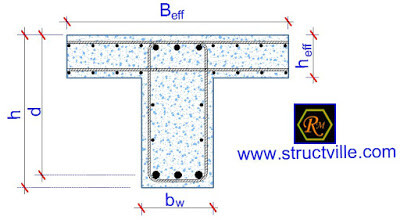# Design of Doubly-Reinforced Beams According to EC2 | Worked ExampleIn the design of reinforced concrete beams, if the design ultimate moment is greater than the ultimate moment of resistance i.e. MEd > MRd, then compression reinforcement is required. Provided that d2/x ≤ 0.38 (i.e. compression steel has yielded) where d2 is the depth of the compression steel from the compression face and x = (d − z)/0.4

The area of compression reinforcement, As2, is given by:

Area of compression reinforcement AS2 = (MEd – MRd) / (0.87fyk (d – d2)) ————- (1)

Area of tension reinforcement As1 = MRd / (0.87fykz) + AS2 ———– (2)
Where z = d[0.5+ √(0.25 – 0.882K’)]
where
K’ = 0.167

DESIGN EXAMPLE
To show how this is done, let us consider the flexural design of support A of the beam loaded as shown below. The depth of the beam is 600mm, and the width is 400mm.
fck = 35 N/mm2 ; fyk = 460 N/mm2; concrete cover = 40mm

Top reinforcement (Hogging moment)
Support A
Effective depth (d) = 600 – 40 – 16 – 10 = 534 mm

MEd = 761.24 KNm

But since the flange is in tension, we use the beam width to calculate the value of k (this applies to all support hogging moments)
k = MEd / (fck bwd2 ) = (761.24 × 106) / (35 × 400 × 5342) = 0.1906

Since k < 0.167, compression is required
Area of compression reinforcement AS2 = (MEd – MRd) / (0.87fyk (d – d2))

MRd = 0.167fckbd2 = (0.167 × 35 × 400 × 5342) × 10-6 = 666.69 KNm

d2 = 40 + 16 + 10 = 66 mm

AS2 = ((761.24 – 666.69) × 106) / (0.87 × 460 × (534 – 66)) = 504.22 mm2
Provide 3X16 Bottom (Asprov = 603 mm2)

Area of tension reinforcement As1 = MRd / (0.87fyk z) + AS2
Where z = d[0.5+ √(0.25 – 0.882K’)]
K’ = 0.167
z = d[0.5+ √((0.25 – 0.882(0.167))] = 0.82d

As1 = MRd / (0.87fyk z) + AS2 = ( 666.69 × 106) / (0.87 × 460 × 0.82 × 534) + 504.22 mm2 = 4308.66 mm2

Provide 6X32mm TOP (ASprov = 4825mm2)